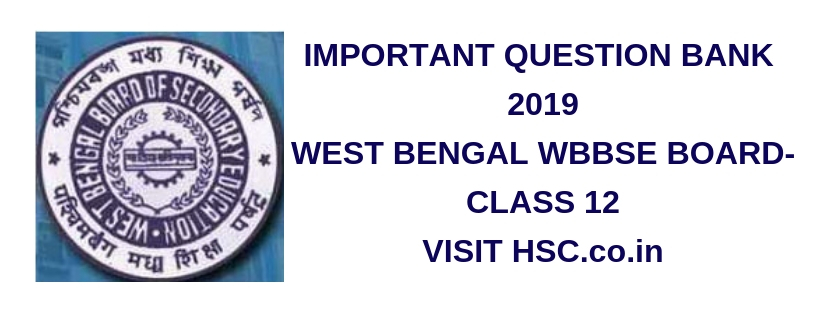# Physics  – Important Question Bank for West Bengal Class XII HS (HSC) Board Exam 2019

HSC Board Exams are fast approaching and students are getting anxious about how to prepare for their HSC Board Exams. So we had mentioned some HSC Study Tips to help students in Cracking HSC Exams.

After the tremendous success of our last year Important Questions Bank for West Bengal Class XII HS (HSC) Board Exam 2016, and 2017we have also created a list of Most Important Question Bank for West Bengal Class XII HS (HSC) Board Exam 2019 which are likely to appear in HSC Board Exams this year.To unlock the content Click on any of 3 buttons available in the box below saying “This content is Locked”. Once you click on the button the content will get unlocked on same page itself. You must click on social media button showing in below box ie Facebook, Twitter or Google Plus to unlock the content.

1. A ball is projected horizontally with a velocity of 5 m/sec from the top of a building 19.6 m high. How long will the ball take to hit the ground?
2. Show that the mechanical energy of a body sliding down smooth inclined plane is conserved.
3. Find a relation between the gravitational constant and mean density of the earth.
4. Write down the relation between pressure and volume of an ideal gas during an adiabatic change and compare the slopes of the isothermal and the adiabatic curves for the gas at a point in the P-V diagram.
5. State that the motion of a simple pendulum is simple harmonic if the amplitude of the pendulum is very small. Obtain an expression for its period of oscillation.
6. Find out the phase difference between two points, 0.3m apart on the path of a travelling wave of wavelength 1m. Show the steps in your working out of the answer.
7. “It is easier to pull a roller obliquely, to move it along the ground, than to push it to do so.” Justify the statement.
8. Name the parameter that decides if a body is in thermal equilibrium with another body or not.
9. State Biot−Savart Law.
10. By using this law find the magnetic induction B at a distance ‘d’ from an infinitely long straight conductor carrying current I.
11. Show that the fundamental frequency of an open pipe is twice that of a closed pipe if they are of same length.
12. Two capacitor of capacitances C1 = 2μF and C2 = 8μF are connected in series and the resulting combination is connected across 300 volt. Calculate the charge, potential difference and energy stored in capacitor separately.
13. Which property of wave shows that sound wave is longitudinal? Calculate the value of Young’s modulus of steel if its density is 7.8 gm/cm3 and if sound travels in it with a velocity of 5200 m/S.
14. An artificial satellite is moving in a circular orbit around the earth with a speed equal to the half of the magnitude of the escape velocity from the earth. Determine the height of the satellite above the Earth surface.
15. What do you mean by centripetal accn? Obtain the expression for centripetal accn of a point particle moving uniformly in a circular path.
16. A metal shows photoelectric effect in green light. Will it show photoelectric effect in violet light? Give reason to your answer.
17. An electron when projected from rest through a potential difference of 60,000 v attains a velocity of 1,46 x 1010 cm/s. Find the ratio of charge and mass of electron.
18. At equal temperature does r.m.s velocity of the molecules of Hydrogen and Oxygen will be same? Give reason.
19. Why centrifugal force is termed as pseudo force?
20. In Fraunhofer diffraction by a single slit, a position where first order minimum is formed by the wave-length of 6000 A, first order maximum is formed due to an unknown wave-length V. Find X’
21. An object is placed at a distance of 25 cm. on the axis of a concave mirror having focal length of 20 cm. Find the image distance, type of the image and its lateral magnification.
22. When a linear object is placed in front of a convex mirror, image of the 4th size of the object is formed. Calculate the object distance and the image distance. This linear object is kept perpendicular to the axis.
23. Explain Analog and Digital Communication.
24. A 900 pF capacitor is charged with the help of 100 V batteries. Calculate the steady electric energy on this capacitor. The above capacitor is disconnected from the battery and is connected to another identical capacitor. What will be the total energy of the system?
25. In Young’s experiment, a beam of light of wave-length 6500 A and 5200 A is used. Find the maximum distance from the central bright fringe, where bright fringes produced by both the wave-length get super-posed. The distance between the two slits is 0.5 mm and the distance between the slits and screen is 100 cm.

Hi, we’re trying to collate and gather the data and would be updating it here a few days before the exam. Please keep on visiting our website for updates.

Please use the comments box below and post questions that you think are important from your analysis. It would help the HSC community a lot.

Do subscribe to our updates so that you do not miss out on any important information that we push your way.

Don’t forget to read : MUST REMEMBER THINGS on the day of Exam for HSC Students